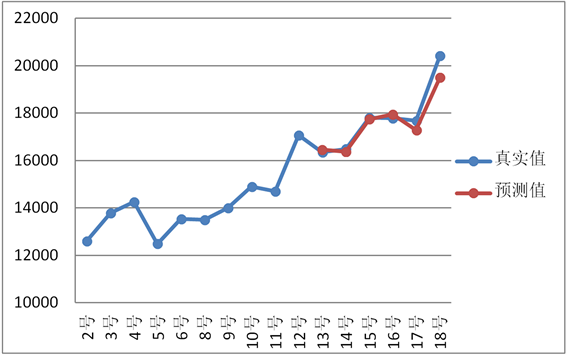# 公共卫生事件信息传播规律模型Information Propagation Model of Public Health Events

DOI: 10.12677/SA.2020.93051, PDF, HTML, XML, 下载: 145  浏览: 436  科研立项经费支持

Abstract: Through the method of combination prediction, this paper studies the phenomenon of information propagation of public health events under the background of new media. By using the grey GM(1,1) model and the weighted Markov chain prediction theory, the grey-weighted Markov chain prediction model is constructed to predict the future trend of public health emergencies, which effectively improves the prediction accuracy. Finally, taking CSFV in Africa as an example, the fea-sibility and validity of the model are verified.

1. 引言

2. 灰色–加权马尔可夫链预测模型

2.1. 灰色预测模型GM(1,1)

${X}^{\left(0\right)}=\left({X}^{\left(0\right)}\left(1\right),{X}^{\left(0\right)}\left(2\right),\cdots ,{X}^{\left(0\right)}\left(n\right)\right)$

${X}^{\left(1\right)}=\left({X}^{\left(1\right)}\left(1\right),{X}^{\left(1\right)}\left(2\right),\cdots ,{X}^{\left(1\right)}\left(n\right)\right)$

${X}^{\left(0\right)}\left(i\right)+a{X}^{\left(1\right)}\left(i\right)=b$ (1)

$\frac{\text{d}{X}^{\left(1\right)}}{\text{d}t}+a{X}^{\left(1\right)}=b$ (2)

$Y=\left[\begin{array}{c}\begin{array}{c}{x}^{\left(0\right)}\left(2\right)\\ {x}^{\left(0\right)}\left(3\right)\end{array}\\ \begin{array}{c}⋮\\ {x}^{\left(0\right)}\left(n\right)\end{array}\end{array}\right]$$B=\left[\begin{array}{cc}-\frac{1}{2}\left({X}^{\left(1\right)}\left(1\right)+{X}^{\left(1\right)}\left(2\right)\right)& 1\\ -\frac{1}{2}\left({X}^{\left(1\right)}\left(2\right)+{X}^{\left(1\right)}\left(3\right)\right)& 1\\ ⋮& ⋮\\ -\frac{1}{2}\left({X}^{\left(1\right)}\left(i-1\right)+{X}^{\left(1\right)}\left(i\right)\right)& 1\end{array}\right]$$i=1,2,\cdots ,n$

$\stackrel{^}{\alpha }={\left({B}^{\text{T}}B\right)}^{-1}{B}^{\text{T}}Y$ (3)

${x}^{\left(1\right)}\left(t\right)=\left({x}^{\left(1\right)}\left(1\right)-\frac{b}{a}\right){\text{e}}^{-at}+\frac{b}{a}$ (4)

${\stackrel{^}{x}}^{\left(1\right)}\left(i+1\right)=\left({x}^{\left(0\right)}\left(1\right)-\frac{b}{a}\right){\text{e}}^{-ak}+\frac{b}{a}$ ; $i=1,2,\cdots ,n$ (5)

${\stackrel{^}{x}}^{\left(0\right)}\left(i+1\right)={\stackrel{^}{x}}^{\left(1\right)}\left(i+1\right)-{\stackrel{^}{x}}^{\left(1\right)}\left(i\right)=\left(1-{\text{e}}^{a}\right)\left({x}^{\left(0\right)}\left(1\right)-\frac{b}{a}\right){\text{e}}^{-ai}$ ; $i=1,2,\cdots ,n$ (6)

2.1.1. 相对关联度检验

${\eta }_{\left(i\right)}$ 为关联系数，根据关联系数定义可得

${\eta }_{\left(i\right)}=\frac{\mathrm{min}\mathrm{min}|{X}^{\left(0\right)}\left(i\right)-{\stackrel{^}{X}}^{0}\left(i\right)|+\rho \mathrm{max}\mathrm{max}|{X}^{\left(0\right)}\left(i\right)-{\stackrel{^}{X}}^{0}\left(i\right)|}{|{\stackrel{^}{X}}^{\left(0\right)}\left(i\right)-{X}^{\left(0\right)}\left(i\right)|+\rho \mathrm{max}\mathrm{max}|{\stackrel{^}{X}}^{\left(0\right)}\left(i\right)-{X}^{\left(0\right)}\left(i\right)|}$ (7)

$\rho$ 为分辨率， $0<\rho <1$，一般取 $\rho =0.5$$\mathrm{min}\mathrm{min}|{X}^{\left(0\right)}\left(i\right)-{\stackrel{^}{X}}^{0}\left(i\right)|$ 为两级最小差， $\mathrm{max}\mathrm{max}|{X}^{\left(0\right)}\left(i\right)-{\stackrel{^}{X}}^{0}\left(i\right)|$ 为两级最大差，令原始序列与预测序列的关联度

$r=\frac{1}{n}{\sum }_{i=1}^{n}\eta \left(i\right)$ (8)

2.2. 马尔可夫链模型

$\epsilon \left(k\right)=\frac{{x}^{\left(0\right)}\left(k\right)-{\stackrel{^}{x}}^{\left(0\right)}\left(k\right)}{{x}^{\left(0\right)}\left(k\right)}$ (9)

${P}^{\left(k\right)}=\left[\begin{array}{cccc}{P}_{11}^{\left(k\right)}& {P}_{12}^{\left(k\right)}& \cdots & {P}_{1n}^{\left(k\right)}\\ {P}_{21}^{\left(k\right)}& {P}_{22}^{\left(k\right)}& \cdots & {P}_{2n}^{\left(k\right)}\\ ⋮& ⋮& \ddots & ⋮\\ {P}_{n1}^{\left(k\right)}& {P}_{n2}^{\left(k\right)}& \cdots & {P}_{nn}^{\left(k\right)}\end{array}\right]$

${P}_{ij}^{\left(k\right)}=\frac{{m}_{ij}^{\left(k\right)}}{{m}_{i}}$ (10)

2.3. 灰色–加权马尔可夫链模型

$P=\left[\begin{array}{cccc}{P}_{11}^{\left(k\right)}& {P}_{12}^{\left(k\right)}& \cdots & {P}_{1n}^{\left(k\right)}\\ {P}_{21}^{\left(k\right)}& {P}_{22}^{\left(k\right)}& \cdots & {P}_{2n}^{\left(k\right)}\\ ⋮& ⋮& \ddots & ⋮\\ {P}_{m1}^{\left(k\right)}& {P}_{m2}^{\left(k\right)}& \cdots & {P}_{mn}^{\left(k\right)}\end{array}\right]$

${P}_{i}={\sum }_{k=1}^{m}{w}_{k}×{p}_{i}^{\left(k\right)},\text{\hspace{0.17em}}i\in S$ (11)

${w}_{k}=\frac{|{r}_{k}|}{{\sum }_{i=1}^{m}|{r}_{k}|}$ (12)

${r}_{k}=\frac{{\sum }_{i=1}^{n-k}\left({x}_{i}-\stackrel{¯}{x}\right)\left({x}_{i+k}-\stackrel{¯}{x}\right)}{\sqrt{{\sum }_{i=1}^{n-k}{\left({x}_{i}-\stackrel{¯}{x}\right)}^{2}{\sum }_{i=1}^{n-k}{\left({x}_{i+k}-\stackrel{¯}{x}\right)}^{2}}}$, $k=1,\cdots ,5$ (13)

$\stackrel{^}{Y}\left(k\right)={\stackrel{^}{x}}^{\left(0\right)}\left(k\right)\left[1+\frac{1}{2}\left({b}_{i}+{a}_{i}\right)\right]$ (14)

3. 案例分析

3.1. GM(1,1)模型对非洲猪瘟事件百度搜索指数的预测和状态划分Table 1. The actual Baidu search index, predicted Baidu Index and relative error status of African swine fever from April

4 to 18

3.2. 加权马尔可夫链预测

${P}^{\left(1\right)}=\left[\begin{array}{ccccc}0& \frac{1}{3}& \frac{1}{3}& \frac{1}{3}& 0\\ \frac{1}{3}& 0& \frac{1}{3}& 0& \frac{1}{3}\\ 0& \frac{1}{3}& 0& \frac{1}{3}& \frac{1}{3}\\ \frac{3}{5}& \frac{1}{5}& 0& \frac{1}{5}& 0\\ 1& 0& 0& 0& 0\end{array}\right]$

${P}^{\left(2\right)}=\left[\begin{array}{ccccc}0& 0& \frac{1}{3}& \frac{2}{3}& 0\\ \frac{1}{3}& \frac{1}{3}& 0& 0& \frac{1}{3}\\ \frac{2}{3}& 0& 0& \frac{1}{3}& 0\\ \frac{1}{4}& 0& \frac{1}{2}& \frac{1}{4}& 0\\ 0& 1& 0& 0& 0\end{array}\right]$

${P}^{\left(3\right)}=\left[\begin{array}{ccccc}\frac{2}{3}& \frac{1}{3}& 0& 0& 0\\ \frac{1}{3}& 0& 0& \frac{2}{3}& 0\\ \frac{1}{2}& \frac{1}{2}& 0& 0& 0\\ 0& 0& \frac{1}{4}& \frac{1}{2}& \frac{1}{4}\\ 0& 0& 1& 0& 0\end{array}\right]$

${P}^{\left(4\right)}=\left[\begin{array}{ccccc}0& 0& \frac{1}{2}& \frac{1}{2}& 0\\ \frac{1}{3}& \frac{1}{3}& 0& \frac{1}{3}& 0\\ 0& 0& \frac{1}{2}& \frac{1}{2}& 0\\ \frac{3}{4}& 0& 0& \frac{1}{4}& 0\\ 0& 1& 0& 0& 0\end{array}\right]$

${P}^{\left(5\right)}=\left[\begin{array}{ccccc}\frac{1}{2}& 0& 0& \frac{1}{2}& 0\\ \frac{1}{3}& 0& \frac{1}{3}& \frac{1}{3}& 0\\ 0& \frac{1}{2}& 0& \frac{1}{2}& 0\\ \frac{1}{3}& \frac{1}{3}& \frac{1}{3}& 0& 0\\ 0& 0& 0& 1& 0\end{array}\right]$Table 3. Autocorrelation coefficient and weight of each orderTable 4. Prediction of relative error state on July 13Figure 1. Forecast result chartTable 6. Comparison table of relative error results

4. 结论

1) 对于有一定波动性的数据来说，通过传统的灰色预测模型GM(1,1)计算得到的百度搜索指数预测值相对误差较大，而马尔可夫链对于波动性大的数据预测较为准确，所以用加权马尔可夫链对模型进行优化，结果表明，构建的灰色–加权马尔可夫链模型能够有效地减小相对误差值，提高模型的精度，使预测结果更具有可信度。

2) 本文对突发公共卫生事件的未来趋势预测提供了一种预测结果较为精确的组合模型，帮助了解突发公共卫生事件发展和影响范围，描述突发公共卫生事件传播规律，对及时辅助决策者做出科学合理的决策行为具有一定指导意义。

  王林, 王可, 吴江. 社交媒体中突发公共卫生事件舆情传播与演变——以2018年疫苗事件为例[J]. 数据分析与知识发现, 2019(4): 42-52.  马天娇. 融媒时代突发公共卫生事件信息传播及风险沟通策略探讨[J]. 新闻研究导刊, 2018, 9(21): 85-86.  张倩生, 谢柏林, 张新猛. 网络舆情突发事件的应急群决策方法[J]. 统计与决策, 2016(10): 38-41.  Galam, S. (2002) Minority Opinion Spreading in Random Geometry. The European Physical Journal B, 25, 403-406. https://doi.org/10.1140/epjb/e20020045  靳松, 庄亚明. 基于H7N9的突发事件信息传播网络簇结构特性研究[J]. 情报杂志, 2013, 32(12): 12-17.  洪巍, 吴林海, 吴祐昕. 食品安全网络舆情中的网络意见领袖[J]. 华南农业大学学报(社会科学版), 2014, 13(4): 101-110.  滕文杰. 时间序列分析法在突发公共卫生事件网络舆情分析中的应用研究[J]. 中国卫生统计, 2014(6): 1071-1073.  刘小洋, 何道兵. 基于突发公共事件的信息传播动力学模型与舆情演化研究[J], 计算机科学, 2019, 46(5): 320-326.  李海晨, 张爱真. 基于灰色理论的科技文献增长规律研究——以微信舆情为例[J]. 情报探索, 2019(2): 7-12.  林元烈. 应用随机过程[M]. 北京: 清华大学出版社, 2002.  乔松珊, 张建军. 基于灰色马尔可夫修正模型的城市月用电需求预测[J]. 电力需求侧管理, 2013, 15(1): 11-14.  蒋峰, 王宗耀, 张鹏. 基于灰色-加权马尔可夫链的光伏发电量预测[J]. 电力系统保护与控制, 2019, 47(15): 55-60.  宋巧娜, 唐德善. 基于灰色马尔可夫模型的农业用水量预测[J]. 安徽农业科学, 2007, 36(6): 1788-1789.